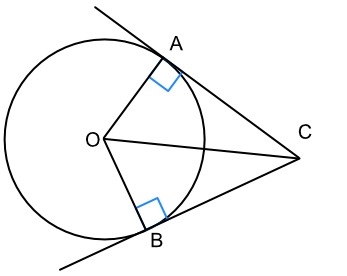Circle Theorems - Tangents

## Circle Theorems - Tangents

A tangent is a line that just touches the circumference of a circle. It can touch at any point on the circumference. At the point of contact, the angle between the tangent and the radius is 90º.In the diagram below, AC and BC are both tangents to a circle. Triangles OAC and BOC are congruent (identical): OC is common to both triangles, and in each case opposite a right angle; and OA and OB are both equal lengths (radius). As the triangles are congruent, AC = BC.

Tangents to a circle drawn to a point outside the circle are equal in length.## Example 1

Give the size of the angle ∠ACB.∠OAC is a right angle; as is ∠OBC.

360 - 90 - 90 - 119 = 61

## Example 2

A, B and D are points on the circumference of a circle with a centre O. AC and BC are tangents to the circle. ∠ACB has an angle of 61º. What is the size of ∠ADB?Angles in a quadrilateral = 360º and ∠OAC and ∠OBC are right angles

∠AOB = 360 - 90 - 90 - 61 = 119º

AOB and ADB are subtended angles on the same chord.

∠ADB = frac(1)(2) ∠AOB

∠ADB = frac(119)(2) = 59.5º